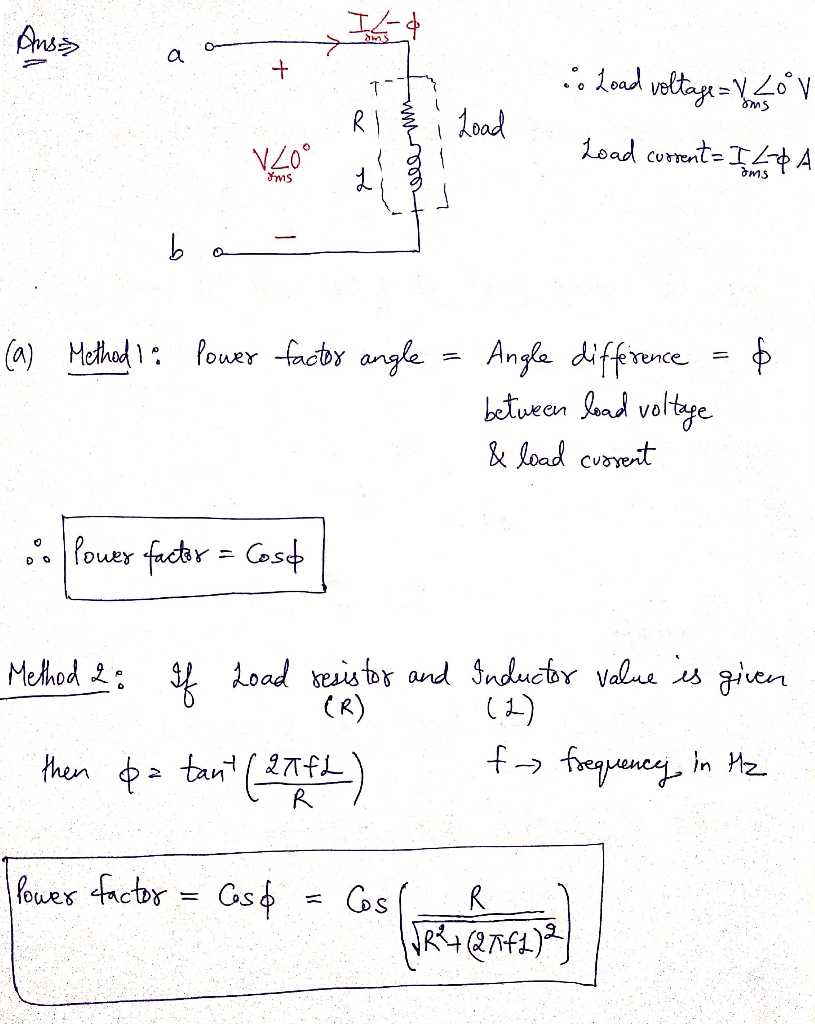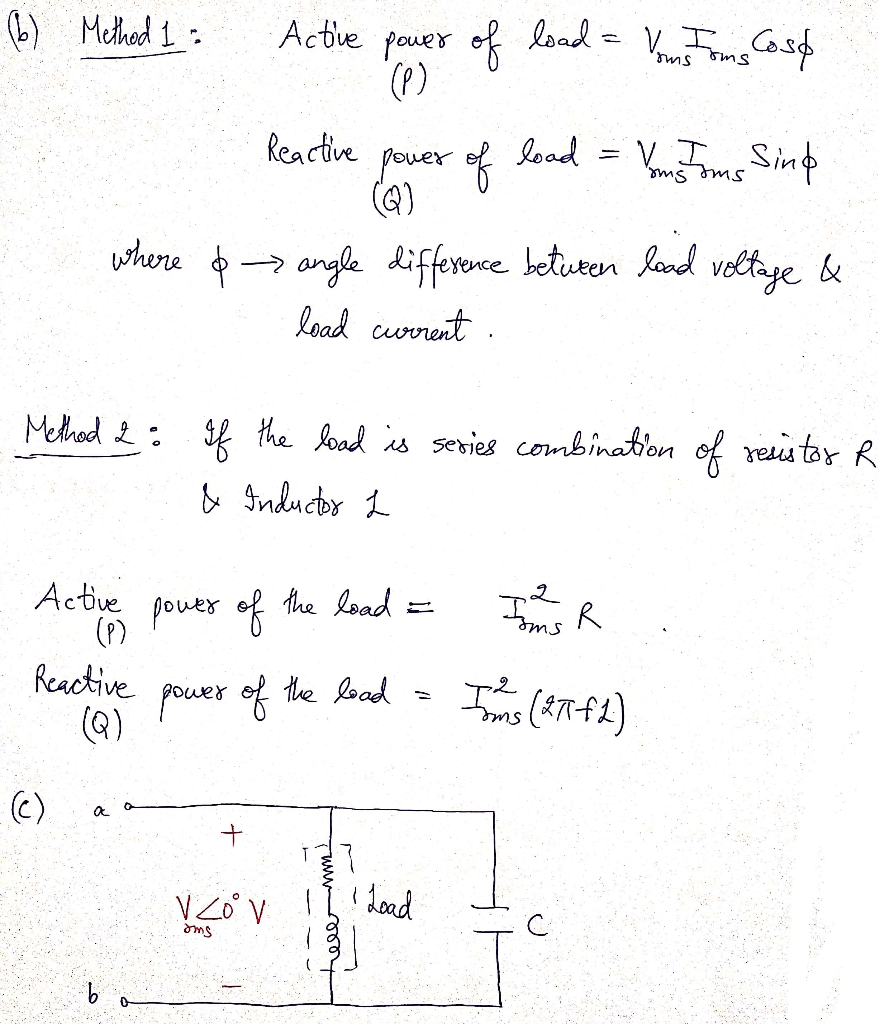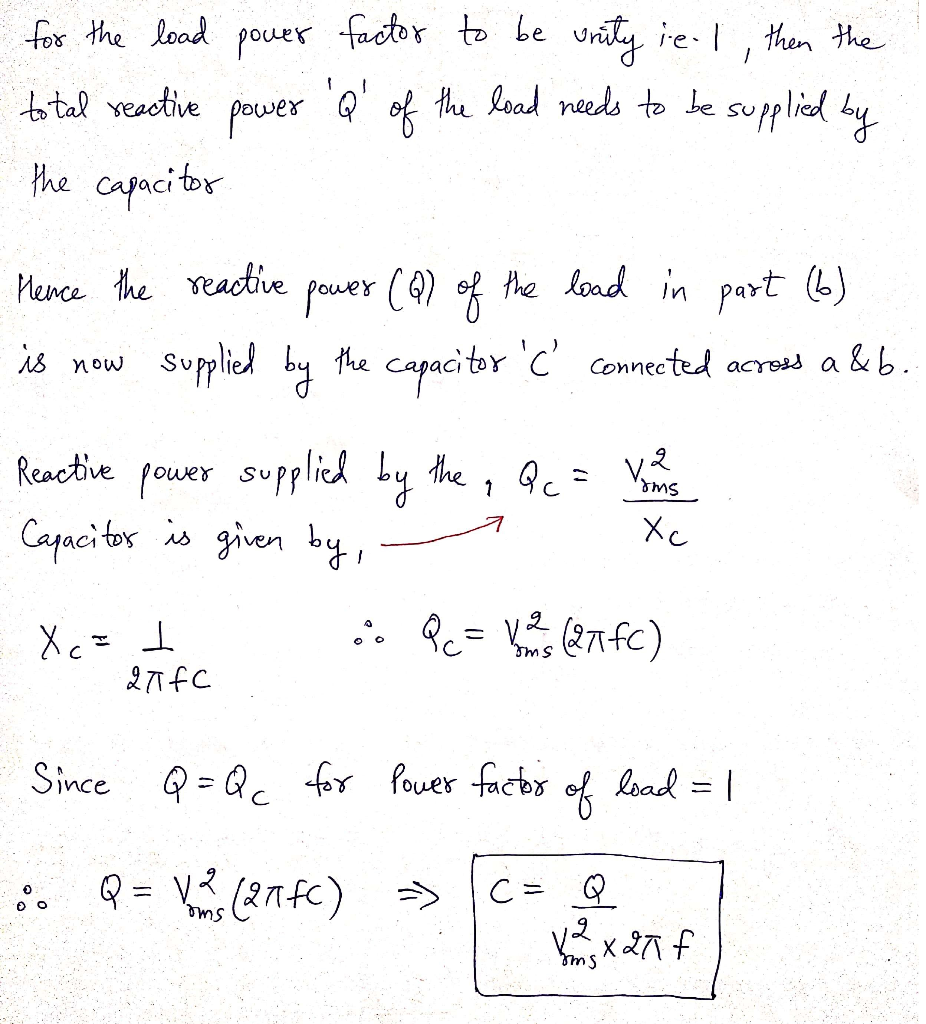##### Question

In: Electrical Engineering

# The power supply frequency is 60Hz. a) What is the power factor of the load? b)...

The power supply frequency is 60Hz.

a) What is the power factor of the load?

b) What is the active power [W] and reactive power [VAR] consumed by the resistors and inductances?

c) Connect capacitor C to points a and b to set the power factor of the entire load to 1. What is the capacitance [F] of the capacitor?

Since there is no picture, just tell me how to solve it.

## Solutions

##### Expert SolutionFeel free to ask if you have any query.Thank you.

## Related Solutions

##### 3. A 60Hz, single phase line transmits power to an inductive load that has a receiving...
3. A 60Hz, single phase line transmits power to an inductive load that has a receiving voltage of 13.8KV, reactive factor of 0.6 and 5 MVA respectively. If this line has a impedance of 1+j2 ohms per conductor, Calculate the following: The voltage at the sending end (10 pts) b. The transmission line efficiency (10 pts) c. If this line is connected in parallel to a telephone wire and the conductors of the power line is labelled a and b...
##### 1. What is the importance of power factor in the supply system ? 2. Why is...
1. What is the importance of power factor in the supply system ? 2. Why is the power factor not more than unity ? 3. What is the effect of low power factor on the generating stations ? 4. Why is unity power factor not the most economical p.f. ? 5. Why a consumer having low power factor is charged at higher rates ?
##### 1) A 25 kVA transformer supplies a load of 12 kW at power factor of 0.6...
1) A 25 kVA transformer supplies a load of 12 kW at power factor of 0.6 lagging. How much kVA can be added before the transformer is at rated load a)when the added load is at 1.0 pf? b)when the added load is at 0.866 pf leading?
##### Design an AC RLC circuit that can supply power to a load which is made up...
Design an AC RLC circuit that can supply power to a load which is made up of an 8 - mu*F capacitor and a resistor with a resistance that can be varied between 600 Q and 800 The average power consumed by the circuit must be 2 kW or less and the power factor must be above 0.95 for all conditions of the load. The frequency of the power supply is 50 Hz. The design should include an inductor and...
##### Design an AC RLC circuit that can supply power to a load which is made up...
Design an AC RLC circuit that can supply power to a load which is made up of an 8 - mu*F capacitor and a resistor with a resistance that can be varied between 600 Q and 800 The average power consumed by the circuit must be 2 kW or less and the power factor must be above 0.95 for all conditions of the load. The frequency of the power supply is 50 Hz. The design should include an inductor and...
##### Question 1 This lab will require a power supply but what kind of power supply? This...
Question 1 This lab will require a power supply but what kind of power supply? This will be very important to the lab as the wrong power supply setting means a correctly assembled circuit will not work. (Note: depending on the edition of the lab manual this information can be found in the Basic Measurement or Simple Circuit lab write-ups.) Group of answer choices AC DC Both Neither. Solar Question 2 What is Ohm's Law?                  ...
##### Wind power generation system design; a-) Adopt Rayleigh wind regime. b-) Specify the power factor of...
Wind power generation system design; a-) Adopt Rayleigh wind regime. b-) Specify the power factor of the wind turbine. c-) Find the start, stop and nominal speed of the wind turbine d-) Calculate the annual energy production of the system
##### Wind power generation system design; a-) Adopt Rayleigh wind regime. b-) Specify the power factor of...
Wind power generation system design; a-) Adopt Rayleigh wind regime. b-) Specify the power factor of the wind turbine. c-) Find the start, stop and nominal speed of the wind turbine d-) Calculate the annual energy production of the system
##### c)    A 3-phase load draws an apparent power of 80kVA from a 415V 3-phase supply. The...
c)    A 3-phase load draws an apparent power of 80kVA from a 415V 3-phase supply. The power factor of the load is 0.85. Determine the real power supplied to the load and the rms line current? D) Explain the importance of energy storage for grids that have large amounts of wind power. Name one type of storage system used within a large electricity grid. E) Explain why AC loads are often rated in terms of their apparent power (VA), rather...
##### A three phase 10kW, 1700rpm, 60Hz, four poles, Y connected induction motor has a no-load speed...
A three phase 10kW, 1700rpm, 60Hz, four poles, Y connected induction motor has a no-load speed of 1750rpm. The rotational mechanical losses are constant and equal to 500W. The core losses are constant and equal to 300W. The copper losses in the stator equal to the copper losses in the rotor at full load. If the rotor resistance per phase is 0.25?, calculate the following: a.The frequency of the induced current in the rotor circuit at full load. b.The percentage...